### The Stark Effect for n=2 Hydrogen.

The Stark effect for the n=2 states of hydrogen requires the use of degenerate state perturbation theory since there are four states with (nearly) the same energies. For our first calculation, we will ignore the hydrogen fine structure and assume that the four states are exactly degenerate, each with unperturbed energy of. That is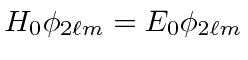. The degenerate states,,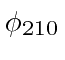, and.

The perturbation due to an electric field in the z direction is. So our first order degenerate state perturbation theory equation isThis is esentially a 4X4 matrix eigenvalue equation. There are 4 eigenvalues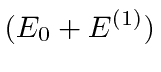, distinguished by the index.

Because of the exact degeneracy,andcan be eliminated from the equation.This is just the eigenvalue equation forwhich we can write out in (pseudo)matrix formNow, in fact, most of the matrix elements ofare zero. We will show that because, that all the matrix elements between states of unequalare zero. Another way of saying this is that the operator z doesn't change''. Here is a little proof.This implies thatunless.

Lets define the one remaining nonzero (real) matrix element to be.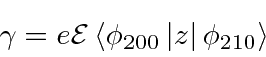The equation (labeled with the basis states to define the order) is.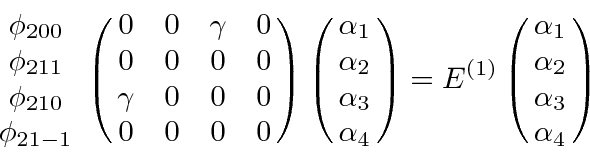We can see by inspection that the eigenfunctions of this operator are,, andwith eigenvalues (of) of 0, 0, and.

What remains is to compute. Recall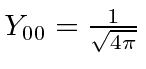and.This is first order in the electric field, as we would expect in first order (degenerate) perturbation theory.

If the states are not exactly degenerate, we have to leave in the diagonal terms of. Assume that the energies of the two (mixed) states are, wherecomes from some other perturbation, like the hydrogen fine structure. (Theandare still not mixed by the electric field.)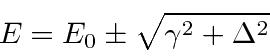This is OK in both limits,, and. It is also correct when the two corrections are of the same order.

Jim Branson 2013-04-22